# Sliding motion along a frictionless circular incline

(diff) ← Older revision | Latest revision (diff) | Newer revision → (diff)
This article discusses a scenario/arrangement whose statics/dynamics/kinematics can be understood using the ideas of classical mechanics.
View other mechanics scenarios

This article describes the sliding motion of a small block placed on a frictionless circular incline. This is similar to the situation of sliding motion along an inclined plane, except that the inclined plane has constant slope whereas in the case of the circular incline, the slope is constantly changing.

The more general version with friction is computationally much harder in terms of its kinematic evolution, and is discussed under sliding motion along a circular incline.

If$\theta$ denotes the angle that the radial line to the circular incline makes with the vertical, then$\theta$ is also the angle made by the tangent line with the horizontal. Locally, the analysis when the block is at such a point on the circular incline looks very similar to the analysis of sliding motion along a frictionless inclined plane where the plane makes an angle of$\theta$ with the horizontal. The key difference is that, since the motion is circular, the normal component of acceleration is not zero; rather, it is given by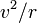$v^2/r$ where$v$ is the speed and$r$ is the radius.

## Basic components of force diagram

A good way of understanding the force diagram is using the coordinate axes as the axis tangent to the circular incline and normal to the circular incline (which in this case is radial). For simplicity, we will assume a two-dimensional situation, with no forces acting along the horizontal axis that is part of the circular incline (in our pictorial representation, this no action axis is the axis perpendicular to the plane).

### The two candidate forces

Assuming no external forces are applied, there are two candidate forces on the block:

Force (letter) Nature of force Condition for existence Magnitude Direction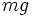$mg$ gravitational force unconditional$mg$ where$m$ is the mass and$g$ is the acceleration due to gravity vertically downward, hence an angle$\theta$ with the normal to the incline and an angle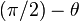$(\pi/2) - \theta$ with the incline$N$ normal force unconditional adjusts so that there is no net acceleration perpendicular to the plane of the incline outward normal to the incline

### Taking components of the gravitational force

The most important thing for the force diagram is understanding how the gravitational force, which acts vertically downward on the block, splits into components along and perpendicular to the incline. The component along the incline is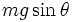$mg\sin \theta$ and the component perpendicular to the incline is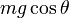$mg\cos \theta$. The process of taking components is illustrated in the adjacent figure

### Component perpendicular to the circular incline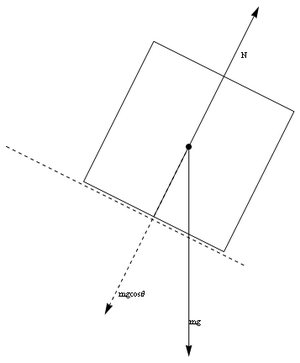Normal component$mg\cos \theta$ of gravitational force$mg$ should cancel out normal force.

In this case, assuming a stable surface of contact, and that the circular incline does not break under the weight of the block, and no other external forces, we get the following equation from Newton's first law of motion applied to the axis perpendicular to the circular incline:$\! N - mg \cos \theta = mv^2/r$

where$N$ is the normal force between the block and the circular incline,$m$ is the mass of the block, and$g$ is the acceleration due to gravity.$N$ acts inward on the circular incline and outward on the block.

### Component along (down) the circular incline

For the axis down the circular incline, the gravitational force component is$mg\sin \theta$. There are no other forces in this direction, so, if$a$ denotes the tangential acceleration (which is also the change in speed) measured positive in the downward direction, we get: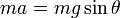$ma = mg \sin \theta$

After cancellation of$m$, we get:$a = g \sin \theta$

Note that if the block is sliding upward (for instance, if given an initial upward velocity) this acceleration functions as retardation, whereas if the block is sliding downward (which may happen if the block is placed at rest, or given an initial downward velocity, or of it turns back after sliding upward) then the acceleration increases the speed.

The overall system of equation is thus: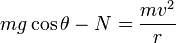$\! mg \cos \theta - N = \frac{mv^2}{r}$

and:$\! a = g\sin \theta$

where we have: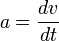$\! a = \frac{dv}{dt}$

## For a block initially placed at rest with radial making angle$\alpha$ with vertical

If the block is placed right on top (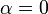$\alpha = 0$), it is in equilibrium, but the equilibrium is an unstable equilibrium, so even a very minor perturbation will cause the block to slide down. We assume that it starts sliding down with a zero initial velocity and deduce the kinematics.

### Speed-position relationship

We have that: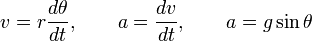$v = r \frac{d\theta}{dt}, \qquad a = \frac{dv}{dt}, \qquad a = g \sin \theta$

Eliminating$t$, we get: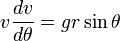$v \frac{dv}{d\theta} = gr \sin \theta$

Integrating, we get that:$v^2 = 2gr(\cos \alpha - \cos \theta)$

or: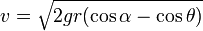$v = \sqrt{2gr(\cos \alpha - \cos \theta)}$

This can also be deduced from energy considerations: the height difference between angles$\alpha$ and$\theta$ is$r(\cos \alpha - \cos \theta)$, so the loss in potential energy is$mgr(\cos \alpha - \cos \theta)$. This becomes kinetic energy, since there is no loss due to friction, and we get: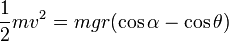$\frac{1}{2}mv^2 = mgr(\cos \alpha - \cos \theta)$

Solve to get$v$ above.

In the case$\alpha = 0$, this becomes: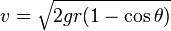$v = \sqrt{2gr(1 - \cos \theta)}$

### Flying-off point

When the speed is sufficiently high, the block flies off the circular incline. To determine this, we need to set$N = 0$. We get:$mg \cos \theta = \frac{mv^2}{r}$

This simplifies to: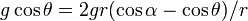$\! g \cos \theta = 2gr(\cos \alpha - \cos \theta)/r$

Cancel the$g$ and$r$ and we get:$\! \cos \theta = 2(\cos \alpha - \cos \theta)$

We get: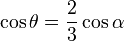$\! \cos \theta = \frac{2}{3}\cos \alpha$

So we get: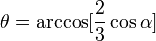$\! \theta = \arccos[\frac{2}{3} \cos \alpha]$

At this point, the speed is: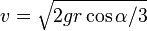$\! v = \sqrt{2gr\cos \alpha/3}$

In particular, when$\alpha = 0$, we get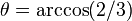$\theta = \arccos(2/3)$ and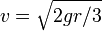$v = \sqrt{2gr/3}$.

### Reintroducing time: kinematics

The time taken to reach from$\alpha$ to$\beta$ can be computed using the following integral:$\! \int_\alpha^\beta \frac{d\theta}{\sqrt{2gr(\cos \alpha - \cos \theta)}} = t$

This integral need not be computable by elementary means, though it is so for some values of$\alpha$. The case$\alpha = 0$ is discussed below:

Fill this in later